Question

# The figure below depicts the aggregate demand curve (AD) and the long-run aggregate supply curve (LRAS) for the United States. The economy is initially at long-run equilibrium, at point A.

The figure below depicts the aggregate demand curve (AD) and the long-run aggregate supply curve (LRAS) for the United States. The economy is initially at long-run equilibrium, at point A.

One of the most contentious issues among economists involves the economy’s adjustment to long-run equilibrium. Some economists believe that adjustment can and should occur naturally. This group, the classical economists, stresses the importance of aggregate supply. Others see the return to long-run equilibrium as an adjustment that occurs unpredictably and often with much delay. This group, the Keynesian economists, stresses the importance of aggregate demand and calls for the government to speed the process back to full employment.

One tenet of classical economics is the assumption that prices are flexible throughout the economy. If prices are completely flexible, then the economy is essentially self-correcting: no matter what factors change in the economy, no matter what curves shift, the economy automatically comes back to full-employment GDP.

Suppose the economy now faces a recession caused by a collapse of the stock market that is also matched by a fall in expected future income. This situation would be represented by a shift of the AD curve to the left, due to a significant drop in consumption expenditure. AD2 is the new AD curve that depicts the fall in consumption expenditure.

According to classical economics, the economy will self-correct and recover from the recession on its own without government intervention. Use the figure below to depict the self-correction of the economy following the leftward shift of the AD curve. Note that this question is specifically asking you to evaluate this scenario as a classical economist. Therefore, you must determine whether you need to drag the curves or whether you need to use the point tool to mark the new equilibrium without shifting any curves.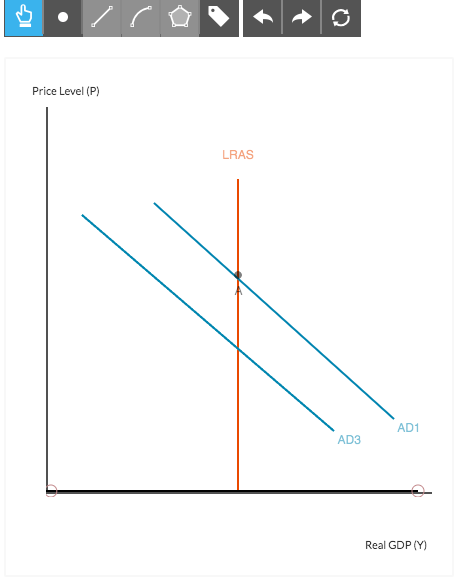I tried shifting AD3 to the left, but was incorrect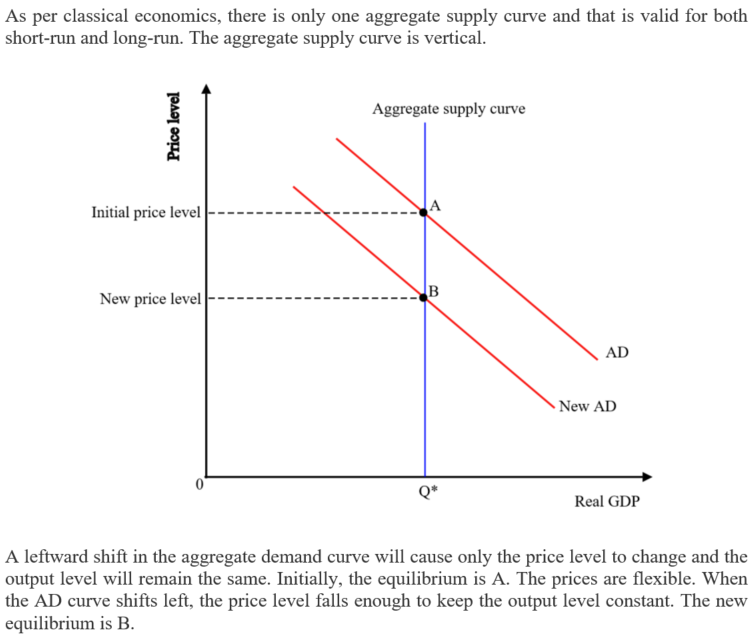#### Earn Coins

Coins can be redeemed for fabulous gifts.

Similar Homework Help Questions
• ### The figure below depicts the aggregate demand curve (AD), the short-run aggregate supply curve (SRAS), and the long-run aggregate supply curve (LRAS) for the United States. The economy is initially at long-run equilibrium, at point A.The figure below depicts the aggregate demand curve (AD), the short-run aggregate supply curve (SRAS), and the long-run aggregate supply curve (LRAS) for the United States. The economy is initially at long-run equilibrium, at point A.One of the most contentious issues among economists involves the economy’s adjustment to long-run equilibrium. Some economists believe that adjustment can and should occur naturally. This group, the classical economists, stress the importance of aggregate supply. Others see the return to long-run equilibrium as an...

• ### The following figure depicts the aggregate demand (AD), theshort-run aggregate supply (SRAS), and the long-run...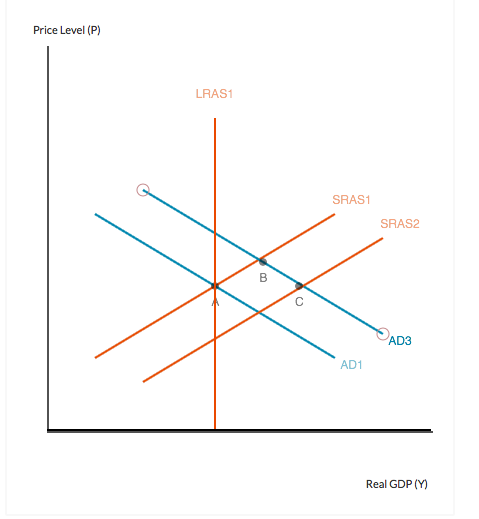The following figure depicts the aggregate demand (AD), the short-run aggregate supply (SRAS), and the long-run aggregate supply (LRAS) curves for an economy. The economy is initially at long-run equilibrium, at point A. Suppose that there is an increase in the amount of investment in the economy due to a reduction in the real interest rate. This increase in investment shifts the AD curve to the right, depicted below in the movement of the economy from point A to point...

• ### Unit 3: Aggregate Demand, Aggregate Supply, and Fiscal Policy AD, AS, and LRAS Short Run vs....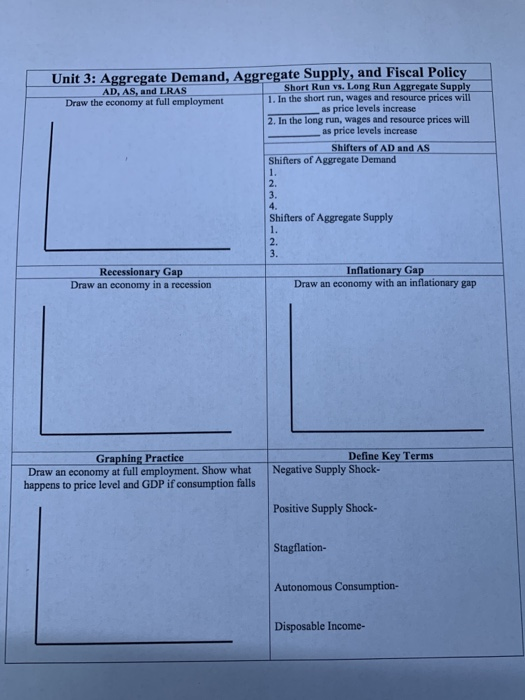Unit 3: Aggregate Demand, Aggregate Supply, and Fiscal Policy AD, AS, and LRAS Short Run vs. Long Run Aggregate Supply Draw the economy at full employment 1. In the short run, wages and resource prices will as price levels increase 2. In the long run, wages and resource prices will as price levels increase Shifters of AD and AS Shifters of Aggregate Demand Shifters of Aggregate Supply imi Recessionary Gap Draw an economy in a recession Inflationary Gap Draw an...

• ### Using aggregate demand (AD), short-run aggregate supply (SRAS) and long-run aggregate supply (LRAS) curves

Question 1: AD-SRAS-LRAS Model Using aggregate demand (AD), short-run aggregate supply (SRAS) and long-run aggregate supply (LRAS) curves, graphically illustrate the effect of an increase in the money supply on output and prices in the short and long run. Assume that the economy is initially in long run equilibrium at the potential output level and prices are fixed in the short-run. In your graph, label "A" for the initial equilibrium, "B' for the short-run equilibrium, and "C" for the long-run equilibrium.

• ### The following graph shows the short-run aggregate supply curve (AS), the aggregate demand curve (AD), and the long-run aggregate supply curve (LRAS) for a hypothetical economy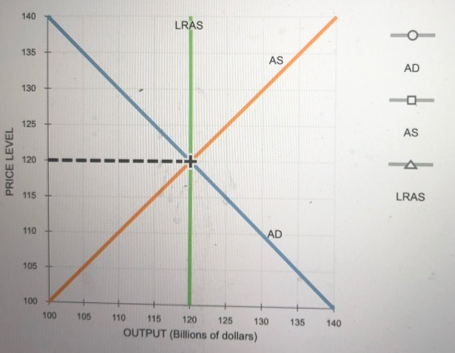9. Economic fluctuations II The following graph shows the short-run aggregate supply curve (AS), the aggregate demand curve (AD), and the long-run aggregate supply curve (LRAS) for a hypothetical economy. Initially, the expected price level is equal to the actual price level, and the economy is in long-run equilibrium at its natural level of output, \$120 billion. Suppose a bout of severe weather drives up agricultural costs, increases the costs of transporting goods and services, and increases the costs of producing goods...

• ### 14 Question (1 point) The following figure depicts the aggregate demand curve (AD), the short-run aggregate...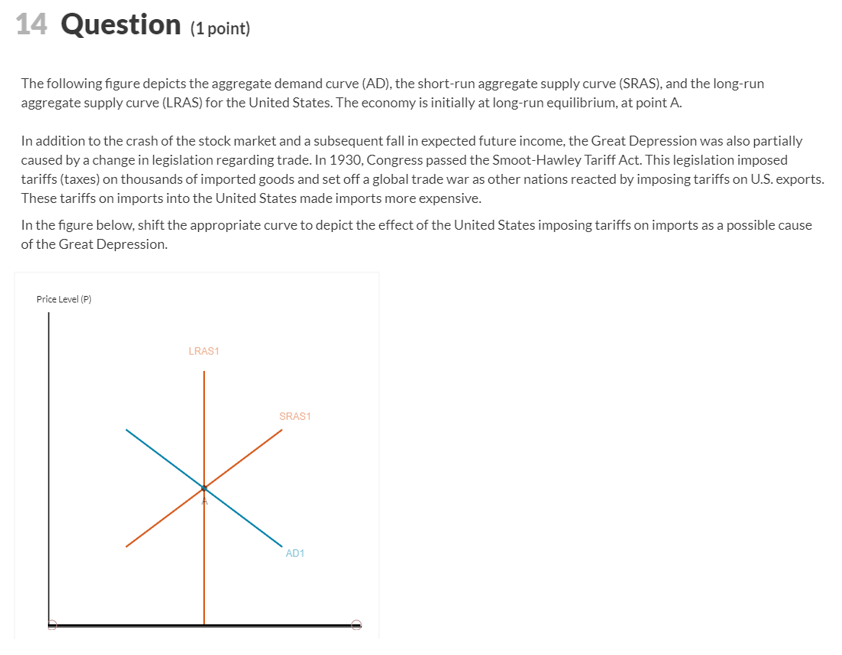14 Question (1 point) The following figure depicts the aggregate demand curve (AD), the short-run aggregate supply curve (SRAS), and the long-run aggregate supply curve (LRAS) for the United States. The economy is initially at long-run equilibrium, at point A. In addition to the crash of the stock market and a subsequent fall in expected future income, the Great Depression was also partially caused by a change in legislation regarding trade. In 1930, Congress passed the Smoot-Hawley Tariff Act. This...

• ### Economics chart The following graph shows the economy in long-run equilibrium at the price level of...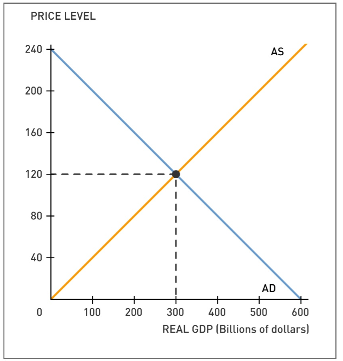Economics chart The following graph shows the economy in long-run equilibrium at the price level of 120 and potential output of \$300 billion. Suppose several foreign economies experience severe recessions, causing foreign purchases of domestic goods and services to decline sharply. Shift the short-run aggregate supply (AS) curve or the aggregate demand (AD) curve to show the short-run impact of the economic turmoil abroad. Tool tip: Click and drag one or both of the curves. Curves will snap into position, so if...

• ### Figure: AD–AS Refer to Figure: AD–AS. Assume that the economy is in long-run equilibrium. If the...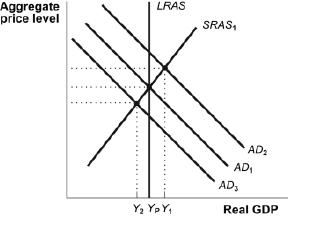Figure: AD–AS Refer to Figure: AD–AS. Assume that the economy is in long-run equilibrium. If the Federal Reserve were to lower the targeted federal funds rate we would most likely expect: there will be a downward movement along the aggregate demand curve AD1. the aggregate demand curve will stay unchanged at AD1. the aggregate demand curve will shift to AD3. the aggregate demand curve will shift to AD2. LRAS Aggregate price level SRAS AD, AD AD, Y₂ YpY, Real GDP

• ### Given a downward-sloping aggregate demand (AD) curve and an upward-sloping short-run aggregate supply curve (SRAS), equilibrium...

Given a downward-sloping aggregate demand (AD) curve and an upward-sloping short-run aggregate supply curve (SRAS), equilibrium occurs where the two intersect. The value on the vertical axis is the equilibrium price level and the value on the horizontal axis is the equilibrium value of real GDP or output. What happens to the economy when AD shifts? It is useful to sketch a graph and show the shift. Suppose, for example, interest rates fall or wealth increases due to a stock...

• ### When the aggregate demand curve and the short-run aggregate supply curve intersect, a) the long-run aggregate...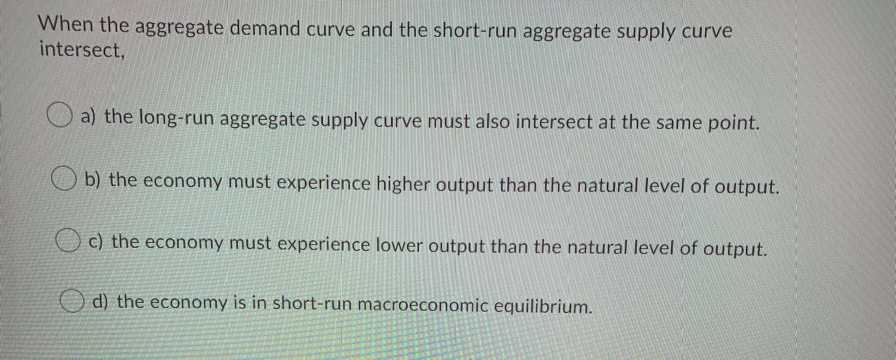When the aggregate demand curve and the short-run aggregate supply curve intersect, a) the long-run aggregate supply curve must also intersect at the same point. Ob) the economy must experience higher output than the natural level of output. o c) the economy must experience lower output than the natural level of output. o d) the economy is in short-run macroeconomic equilibrium. In a small economy in 2016, aggregate expenditure was \$900 million while GDP that year was \$750 million. Which...